# Wheels

Small tractor wheel with a diameter of 60 cm must be rotated 8 times to overcome some pathway. How many times must turn the big tractor wheel with a radius 60 cm to overcome the same distance?

Correct result:

n =  4

#### Solution: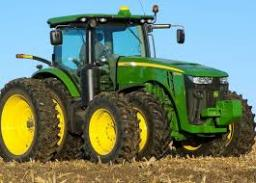We would be pleased if you find an error in the word problem, spelling mistakes, or inaccuracies and send it to us. Thank you!Tips to related online calculators
Check out our ratio calculator.

#### You need to know the following knowledge to solve this word math problem:

We encourage you to watch this tutorial video on this math problem:

## Next similar math problems:

• Wheel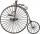Diameter of motocycle wheel is 52 cm. How many times rotates wheel on roand long 2 km?
• WellRope with a bucket is fixed on the shaft with the wheel. The shaft has a diameter 50 cm. How many meters will drop bucket when the wheels turn 15 times?
• Two circlesTwo circles with a radius 4 cm and 3 cm have a center distance 0.5cm. How many common points have these circles?
• Circumference - a simple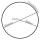What is the ratio of the circumference of any circle and its diameter? Write the result as a real number rounded to 2 decimal places.
• FlowerbedIn the park there is a large circular flowerbed with a diameter of 12 m. Jakub circulated him ten times and the smaller Vojtoseven times. How many meters each went by and how many meters did Jakub run more than Vojta?
• Circle r,DCalculate the diameter and radius of the circle if it has length 26.59 cm.
• MineWheel in traction tower has a diameter 4 m. How many meters will perform an elevator cabin if wheel rotates in the same direction 89 times?
• Wheel diameter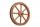A 1m diameter wheel rolled along a 100m long track. How many times did it turn?
• Find theFind the radius of the circular base of the vessel, whose perimeter is 2.51 m. Write the result to one decimal place in meters. Via the π sign - Ludolph's number
• Tree trunk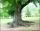Calculate the average tree trunk with a circumference of 149 cm.
• CircleWhat is the radius of the circle whose perimeter is 6 cm?
• DonutsFind how many donuts each student will receive if you share 126 donuts in a ratio of 1:5:8
• Clock handsThe second hand has a length of 1.5 cm. How long does the endpoint of this hand travel in one day?
• Circle - simpleCalculate the area of a circle in dm2, if its circumference is 31.4 cm.
• Regular octagonDraw the regular octagon ABCDEFGH inscribed with the circle k (S; r = 2.5 cm). Select point S' so that |SS'| = 4.5 cm. Draw S (S '): ABCDEFGH - A'B'C'D'E'F'G'H'.
• The pigletThe weight of the piglet grew regularly by five kilograms for four months. Determine the proportion by weight of the piglet in each month, if the first weighed 35 kg.
• 1.5 divided1.5 divided by 1 = w divided by 4